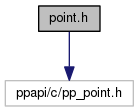Pepper_42_C++_interfaces
point.h File Reference
`#include "ppapi/c/pp_point.h"`
Include dependency graph for point.h:Go to the source code of this file.

## Classes

class  pp::Point
A 2 dimensional point with 0,0 being the upper-left starting coordinate. More...

class  pp::FloatPoint

## Namespaces

pp
The C++ interface to the Pepper API.

## Functions

bool operator== (const pp::Point &lhs, const pp::Point &rhs)

bool operator!= (const pp::Point &lhs, const pp::Point &rhs)

bool operator== (const pp::FloatPoint &lhs, const pp::FloatPoint &rhs)

bool operator!= (const pp::FloatPoint &lhs, const pp::FloatPoint &rhs)

## Detailed Description

This file defines the API to create a 2 dimensional point.

Definition in file point.h.

## Function Documentation

 bool operator!= ( const pp::Point & lhs, const pp::Point & rhs )
inline

Determines whether two Points have different coordinates.

Parameters
 [in] lhs The Point on the left-hand side of the equation. [in] rhs The Point on the right-hand side of the equation.
Returns
true if the coordinates of lhs are equal to the coordinates of rhs, otherwise false.

Definition at line 311 of file point.h.

 bool operator!= ( const pp::FloatPoint & lhs, const pp::FloatPoint & rhs )
inline

Determines whether two Points have different coordinates.

Parameters
 [in] lhs The Point on the left-hand side of the equation. [in] rhs The Point on the right-hand side of the equation.
Returns
true if the coordinates of lhs are equal to the coordinates of rhs, otherwise false.

Definition at line 332 of file point.h.

 bool operator== ( const pp::Point & lhs, const pp::Point & rhs )
inline

Determines whether the x and y values of two Points are equal.

Parameters
 [in] lhs The Point on the left-hand side of the equation. [in] rhs The Point on the right-hand side of the equation.
Returns
true if they are equal, false if unequal.

Definition at line 300 of file point.h.

References pp::Point::x(), and pp::Point::y().

 bool operator== ( const pp::FloatPoint & lhs, const pp::FloatPoint & rhs )
inline

Determines whether the x and y values of two FloatPoints are equal.

Parameters
 [in] lhs The Point on the left-hand side of the equation. [in] rhs The Point on the right-hand side of the equation.
Returns
true if they are equal, false if unequal.

Definition at line 321 of file point.h.

References pp::FloatPoint::x(), and pp::FloatPoint::y().